# Test: Transfer Function

## 20 Questions MCQ Test GATE Electrical Engineering (EE) 2023 Mock Test Series | Test: Transfer Function

Description
Attempt Test: Transfer Function | 20 questions in 60 minutes | Mock test for Electronics and Communication Engineering (ECE) preparation | Free important questions MCQ to study GATE Electrical Engineering (EE) 2023 Mock Test Series for Electronics and Communication Engineering (ECE) Exam | Download free PDF with solutions
QUESTION: 1

Solution:
QUESTION: 2

### Which of the following is not the feature of modern control systems?

Solution:

For a good control system the speed of response and stability must be high and for the slow and sluggish response is not used and undesirable.

QUESTION: 3

### The principles of homogeneity and superposition are applied to ______.

Solution:

Superposition theorem states that for two signals additivity and homogeneity property must be satisfied and that is applicable for the LTI systems.

QUESTION: 4

The sum of the gains of the feedback paths in the signal flow graph shown in fig.  is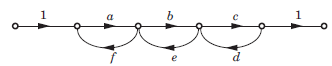Solution:
QUESTION: 5

A control system whose step response is -0.5(1+e-2t) is cascaded to another control block whose impulse response is e-t. What is the transfer function of the cascaded combination?

Solution:
• Let h1(t) is the impulse response of system.
h1(t) = d/dt(-0.5(1+e-2t)) h1(t) = e(-2t) h2(t)  = e(-t)
• Cascading of two system equals to the convolution of both and convolution in time domain is equals to multiplication in frequency domain.
• So by taking Laplace transform of above two
H1(s) =1/(s+2), H2(s) = 1/(s+1)
• Hence the transfer function of cascaded system is
H(s) = H1(s)H2(s)
H(s)= 1/(s+1)(s+2)
QUESTION: 6

The overall transfer function C/R of the system shown in fig. will be: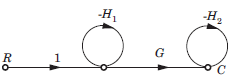Solution: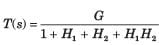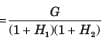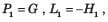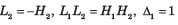QUESTION: 7

Consider the signal flow graphs shown in fig. The transfer 2 is of the graph: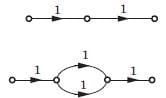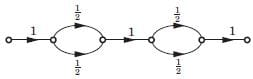Solution:

There are no loop in any graph. So option (B) is correct.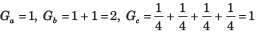QUESTION: 8

Consider the List I and List II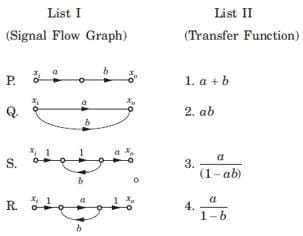The correct match is

Solution:

P. P1 = ab, Δ = 1, L = 0 ,T = ab
Q1 P1 = a, P2 = 6 , Δ = 1, L = Δk = 0,T  = a+b
R. P1 = a, L1 = b, Δ = 1 - b, Δ1 =1,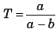S. P1 = a, L1 = ab, Δ = 1 - ab, Δ1 = 1,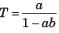QUESTION: 9

For the signal flow graph shown in fig. an equivalent graph is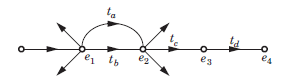Solution:
QUESTION: 10

Consider the block diagram shown in figure.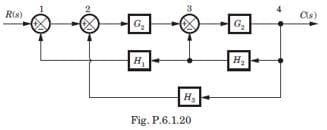For this system the signal flow graph is

Solution:

Option (A) is correct. Best method is to check the signal flow graph. In block diagram there is feedback from 4 to 1 of gain - H1H2 . The signal flow graph of option (A) has feedback from 4 to 1 of gain - H1H2

QUESTION: 11

The block diagram of a system is shown in fig. The closed loop transfer function of this system is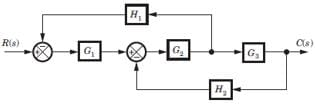Solution:

Consider the block diagram as SFG. There are two feedback loop -G1G2H1 and -G2G3H2 and one forward path G1G2 G3 . So (D) is correct option.

QUESTION: 12

For the system shown in fig. transfer function C(s) R(s) is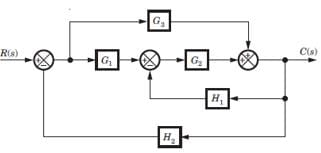Solution:

Consider the block diagram as a SFG. Two forward path G1G2 and G3 and three loops -G1G2 H2, -G2H1, -G3 H2
There are no nontouching loop. So (B) is correct.

QUESTION: 13

In the signal flow graph shown in fig. the transfer function is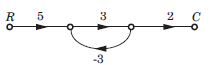Solution:

P1 = 5 x 3 x 2 = 30, Δ = 1 - (3x - 3) = 10
Δ1 = 1,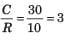QUESTION: 14

In the signal flow graph shown in fig. the gain C/R is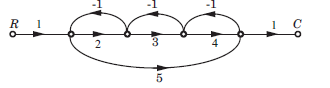Solution:

P1 = 2 x 3 x 4 = 24 , P2 = 1 x 5 x 1 = 5
L1 = -2, L2 = -3, L3 = -4, L4 = -5,
L1L3 = 8, Δ = 1 -(-2 - 3 - 4 - 5) + 8 = 23, Δ1 = 1, Δ2 = 1 - (-3) = 4,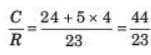QUESTION: 15

The gain C(s)/R(s) of the signal flow graph shown in fig.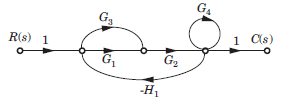Solution:
QUESTION: 16

The negative feedback closed-loop system was subjected to 15V. The system has a forward gain of 2 and a feedback gain of 0.5. Determine the output voltage and the error voltage.

Solution:

Given:
G(s) = 2
H(s) = 0.5 and R(s) = 10V
Output voltage: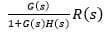= (2/1 + 2 x 0.5) x 15 = 15V
Error voltage: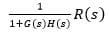= (1/1 + 2 x 0.5) x 15 = 7.5V

QUESTION: 17

For the block diagram shown in fig. transfer function C(s)/R(s) is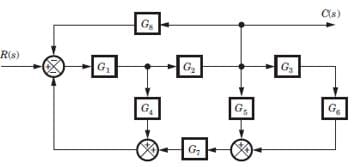Solution:

Four loops -G1G4, -G1G2G5, -G1,G2G5G7 and -G1G2G3G3G7.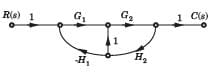There is no nontouching loop. So (B) is correct.

QUESTION: 18

For the block diagram shown in fig. the numerator of transfer function is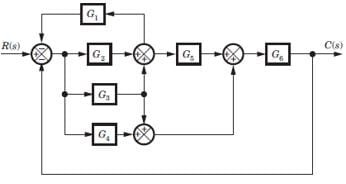Solution:

SFG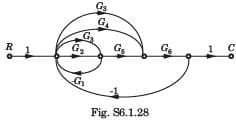P1 = G2G5G6 , P2 = G3G5G6, P3 = G3G6 , P4 = G4G6
If any path is deleted, there would not be any loop.
Hence Δ1 = Δ2 = Δ3 = Δ4 = 1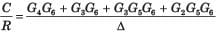QUESTION: 19

For the block diagram shown in fig.  the transfer function C(s)/R(s) is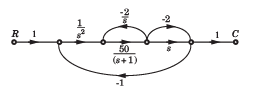Solution: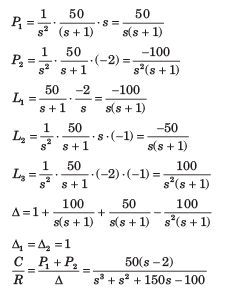QUESTION: 20

In the signal flow graph of figure y/x equals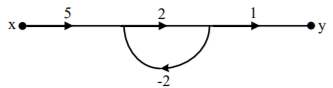Solution:

Transfer function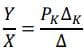PK = 5 x 2 x 1 = 10
ΔK = 1
Δ = 1 - (-4) = 5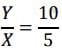= 2Use Code STAYHOME200 and get INR 200 additional OFF Use Coupon Code